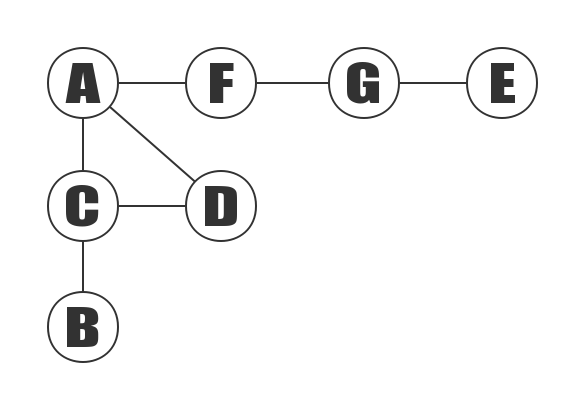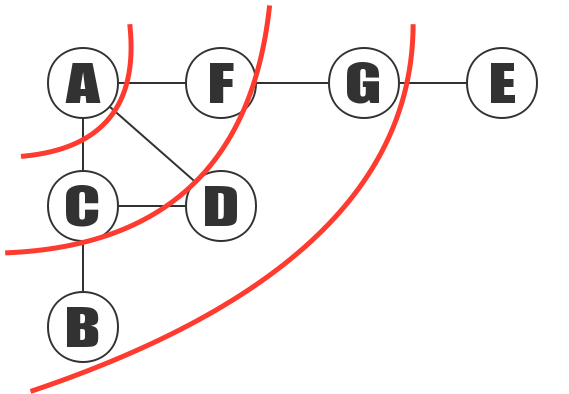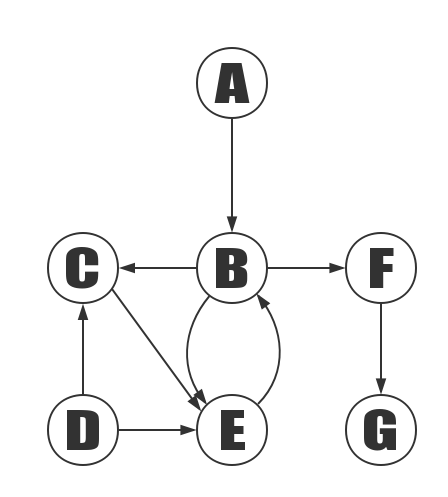## Overview

The breadth first search algorithm, also known as “width first search” or “horizontal priority search”, is called BFS.

From a vertex in the graph, each of the not visited adjacent points of the V is accessed in turn after accessing the starting vertex, then the adjacency points are accessed in turn from these adjacent points, and the adjacent points of the vertex to be accessed are accessed before the adjacent points of the vertices that are accessed, until all the adjacent points of the vertices are accessed. If the vertices are not accessed at this time, we need to select another vertex which has never been visited as a new starting point, repeat the above process until all the vertices in the graph are accessed.

In other words, the process of breadth first search is the starting point and from near to far, access to the path and the path length of the starting vertex and the path length of 1,2…

## Diagram

### Breadth first search for undirected graphs

The following is an example of undirected graph, to demonstrate the depth-first search.Breadth first search of the graph above, starting with vertex A:#### Steps:

2. Access C, D, F in turn. After visiting A, next visit A’s adjoining point. the vertices A,B,C,D,E,F,G are stored in order, so first visit C, after visiting C, next to visit D and F.
3. Access B, G, in turn. After C, D, and F are accessed in the second step, then their adjacency points are accessed in turn. Next first access the adjacency point B of C, and then access the adjacency point G of the F.

### Breadth first search for undirected graphs

The following is an example of directed graph, to demonstrate the Breadth first search.Breadth first search of the graph above, starting with vertex A: